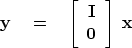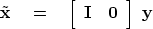Next: Product of operators Up: FAMILIAR OPERATORS Previous: Transient convolution

## Zero padding is the transpose of truncation.

Surrounding a dataset by zeros (zero padding) is adjoint to throwing away the extended data (truncation). Let us see why this is so. Set a signal in a vector, and then make a longer vectorby adding some zeros at the end of.This zero padding can be regarded as the matrix multiplication(3)
The matrix is simply an identity matrixabove a zero matrix.To find the transpose to zero padding, we now transpose the matrix and do another matrix multiply:(4)
So the transpose operation to zero padding data is simply truncating the data back to its original length.Next: Product of operators Up: FAMILIAR OPERATORS Previous: Transient convolution
Stanford Exploration Project
10/21/1998